# A beaker is filled with 200.0 mL of a sodium hydroxide solution with an unknown concentration....

A beaker is filled with 200.0 mL of a sodium hydroxide solution with an unknown concentration. A 0.0100 M solution of HCl is used in the titration. The equivalence point is reached when 18.5 mL of HCl have been added.

What is the initial concentration of NaOH in the beaker?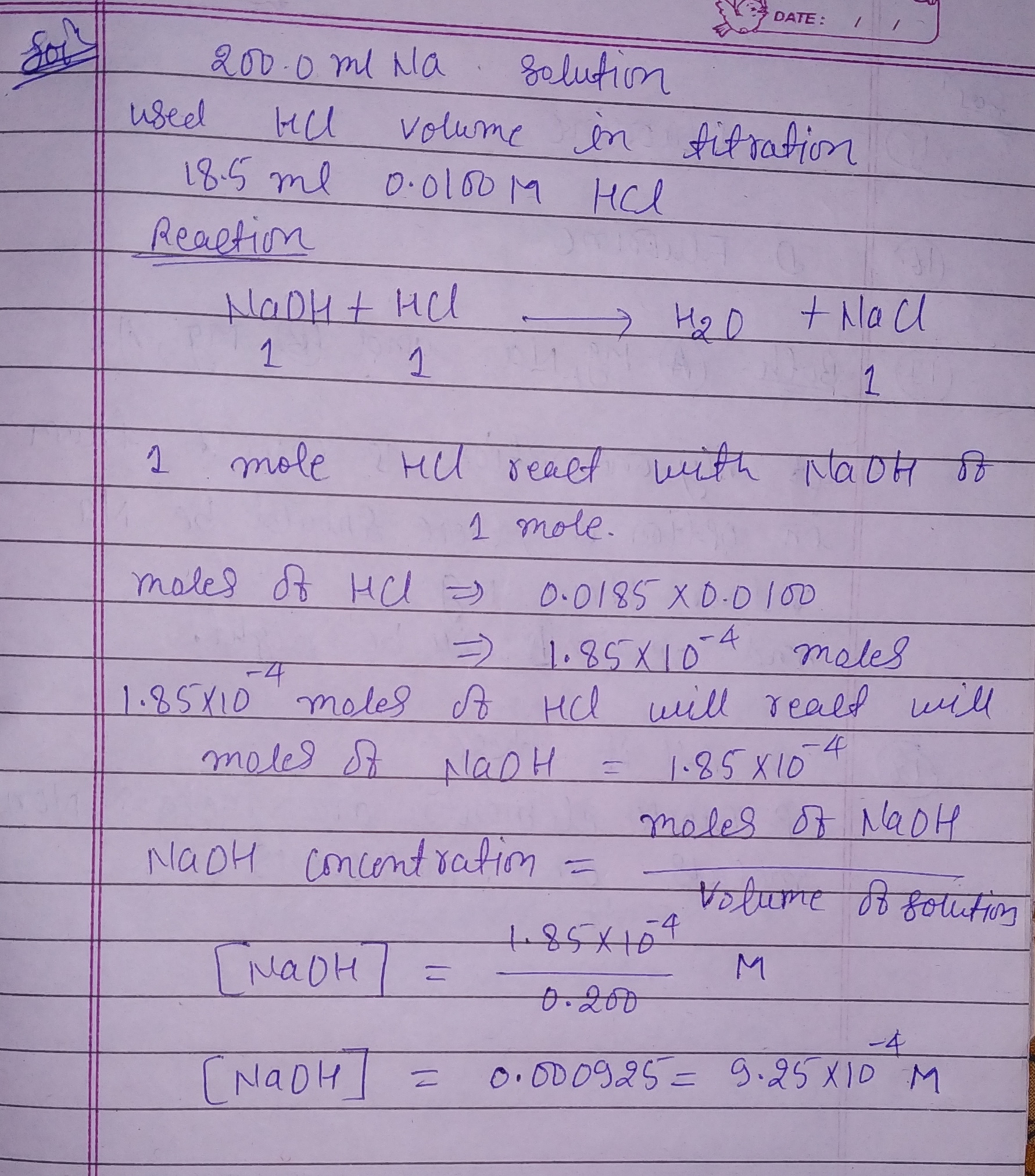#### Earn Coin

Coins can be redeemed for fabulous gifts.

Similar Homework Help Questions
• ### 09 Question (1 point) A beaker is filled with 175.0 mL of a sodium Hydroxide solution...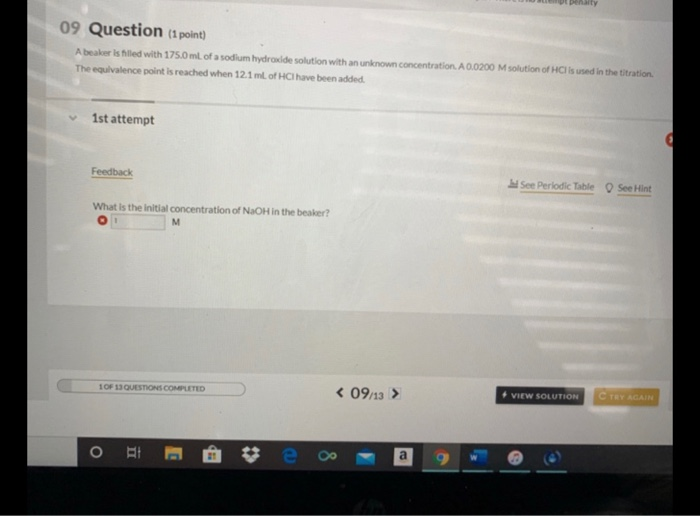09 Question (1 point) A beaker is filled with 175.0 mL of a sodium Hydroxide solution with an unknown concentration. A 0.0200 M solution of His used in the titration The equivalence point is reached when 12.1 ml of HCl have been added 1st attempt Feedback See Periodic Table See Hint What is the initial concentration of NaOH in the beaker? M 1 OF 13 QUESTIONS COMPLETED < 09/13 > • VIEW SOLUTION C TRY AGAIN O TI E

• ### 09 Question (1 point) A beaker is filled with 175.0 ml of a sodium hydroxide solution...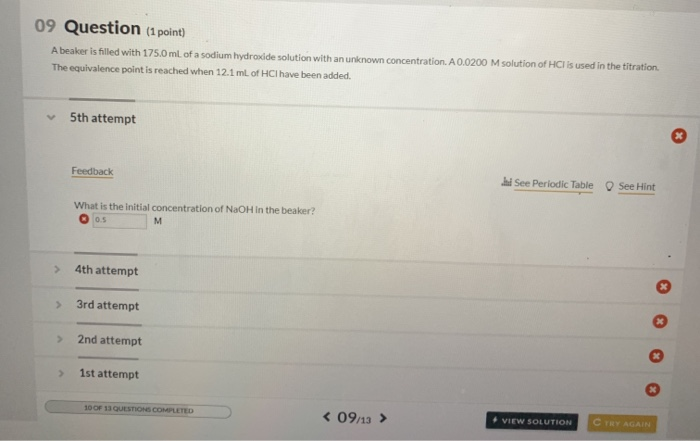09 Question (1 point) A beaker is filled with 175.0 ml of a sodium hydroxide solution with an unknown concentration. A 0.0200 M solution of HCI is used in the titration The equivalence point is reached when 12.1 ml of HCI have been added. 5th attempt Feedback See Periodic Table See Hint What is the initial concentration of NaOH in the beaker? 0.5 M 4th attempt > 3rd attempt > 2nd attempt 1st attempt 10 OF 11 QUESTIONS COMPLETED <...

• ### 1. This is a Numeric Entry question / It is worth 1 point/ You have unlimited...1. This is a Numeric Entry question / It is worth 1 point/ You have unlimited attempts / There is no attempt penalty 09 Question (1 point) A beaker is filled with 175.0 mL of a sodium hydroxide solution with an unknown concentration. A 0.0200 M solution of HCl is used in the titration, The equivalence point is reached when 12.1 mL of HCl have been added. 1st attempt Feedback See Periodic Table See Hint What is the initial concentration...

• ### Part A When titrated with a 0.1198 M solution of sodium hydroxide, a 58.00 mL solution...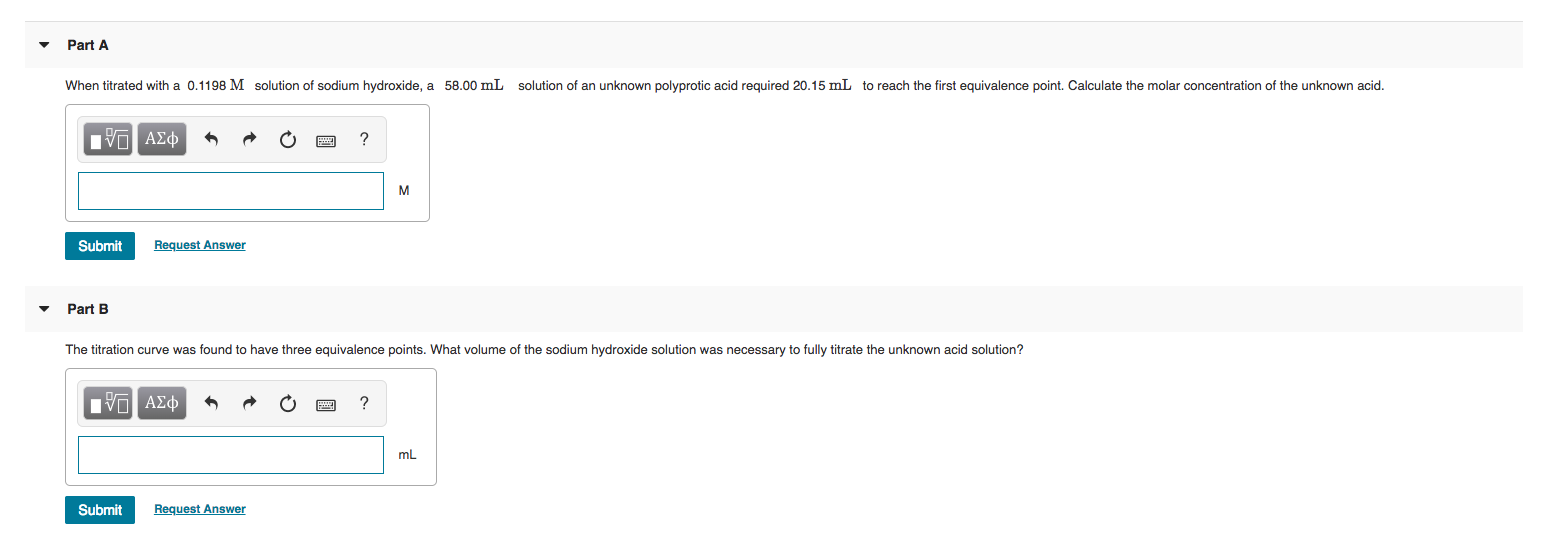Part A When titrated with a 0.1198 M solution of sodium hydroxide, a 58.00 mL solution of an unknown polyprotic acid required 20.15 mL to reach the first equivalence point. Calculate the molar concentration of the unknown acid. O A¢ * R O ? Submit Request Answer Part B The titration curve was found to have three equivalence points. What volume of the sodium hydroxide solution was necessary to fully titrate the unknown acid solution? IVO ADD A O O...

• ### The titration of 23.30 mL of HCl solution of unknown concentration requires 13.00 mL of a...

The titration of 23.30 mL of HCl solution of unknown concentration requires 13.00 mL of a 0.200 M NaOH solution to reach the equivalence point. What is the concentration of the unknown HCl solution in M? Express your answer in moles per liter to three significant figures. M(HCl)M(HCl) = _____________

• ### ACIDS AND BASES Calculating the pH at equivalence of a titration A chemist titrates 200.0 mL...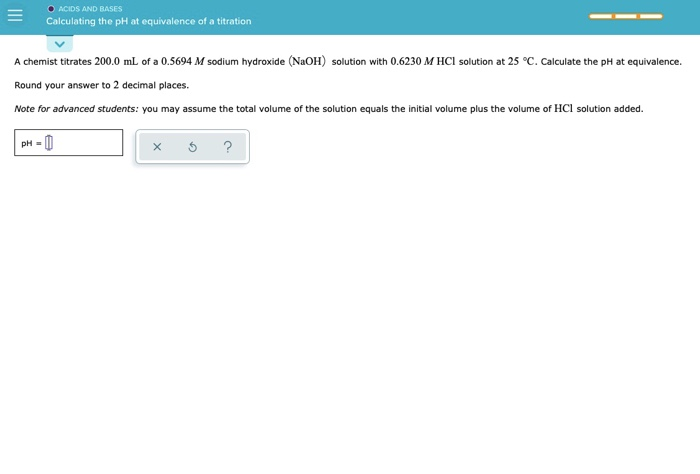ACIDS AND BASES Calculating the pH at equivalence of a titration A chemist titrates 200.0 mL of a 0.5694 M sodium hydroxide (NaOH) solution with 0.6230 M HCl solution at 25 °C. Calculate the pH at equivalence. Round your answer to 2 decimal places. Note for advanced students: you may assume the total volume of the solution equals the initial volume plus the volume of HCl solution added. PH-0 5 ?

• ### 6. Titration of a 50.00 mL solution of an unknown diprotic acid required 35.95 mL of...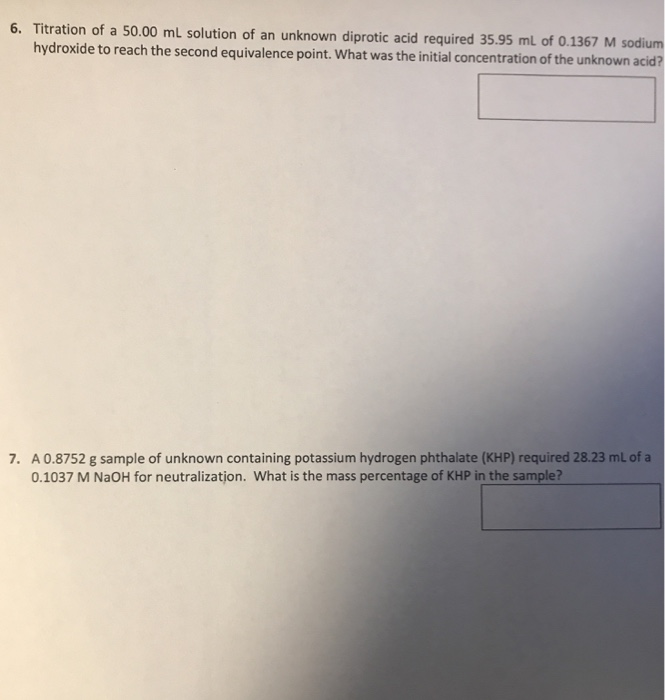6. Titration of a 50.00 mL solution of an unknown diprotic acid required 35.95 mL of 0.1367 M sodium hydroxide to reach the second equivalence point. What was the initial concentration of the unknown acid . A 0.8752 g sample of unknown containing potassium hydrogen phthalate (KHP) required 28.23 mL of a 0.1037 M NaOH for neutralizatjon. What is the mass percentage of KHP in the sample?

• ### An acid-base titration is performed: 250.0 mL of an unknown concentration of HCl(aq) is titrated to...

An acid-base titration is performed: 250.0 mL of an unknown concentration of HCl(aq) is titrated to the equivalence point with 36.7 mL of a 0.1000 M aqueous solution of NaOH. Which of the following statements is not true of this titration? A. At the equivalence point, the OH−concentration in the solution is 3.67×10−3 M. B. The pH is less than 7 after adding 25 mL of NaOH solution. C. The pH at the equivalence point is 7.00. D. The HCl...

• ### Part A The titration of 23.40 mL of HCl solution of unknown concentration requires 12.18 mL...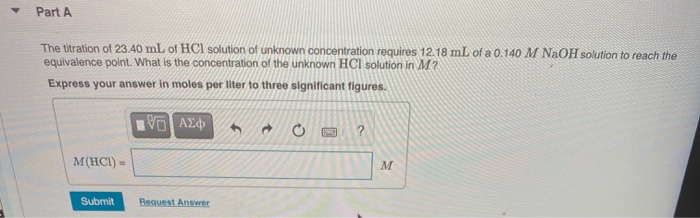Part A The titration of 23.40 mL of HCl solution of unknown concentration requires 12.18 mL of a 0.140 M NaOH solution to reach the equivalence point. What is the concentration of the unknown HCl solution in M? Express your answer in moles per liter to three significant figures. We ΑΣΦ ? M(HCI) M Submit Request Answer

• ### A sodium hydroxide solution of unknown concentration is titrated against 0.8765 g KHPh (Potassium acid phalate, a monoprotic acid, MW 204.3 g/mol)

1.A sodium hydroxide solution of unknown concentration is titrated against 0.8765 g KHPh (Potassium acid phalate, a monoprotic acid, MW 204.3 g/mol). 48.6 mL of the sodium hydroxide solution is required to reach the endpoint of the titration. What is the concentration of the unknown sodium hydroxide solution. 2.This sodium hydroxide solution is then used to titrate an unknown nitric acid sample. 25.0 mL of the nitric acid solution is titrated. 32.8 mL of the sodium hydroxide solution is required...